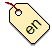# Addison Phillips Java regexp for language tags

Here is a regular expression to parse the future versions of language tags. Suitable for the syntax of the RFC 5646. Written by Addison Phillips, `addison - at - amazon.com` for the Java programming language.

```     static final String langtag_ex =
"(\\A[xX]([\\x2d]\\p{Alnum}{1,8})*\\z)"
+ "|(((\\A\\p{Alpha}{2,8}(?=\\x2d|\\z)){1}"
+ "(([\\x2d]\\p{Alpha}{3})(?=\\x2d|\\z)){0,3}"
+ "([\\x2d]\\p{Alpha}{4}(?=\\x2d|\\z))?"
+ "([\\x2d](\\p{Alpha}{2}|\\d{3})(?=\\x2d|\\z))?"
+ "([\\x2d](\\d\\p{Alnum}{3}|\\p{Alnum}{5,8})(?=\\x2d|\\z))*)"
+ "(([\\x2d]([a-wyzA-WYZ](?=\\x2d))([\\x2d](\\p{Alnum}{2,8})+)*))*"
+ "([\\x2d][xX]([\\x2d]\\p{Alnum}{1,8})*)?)\\z";
```